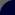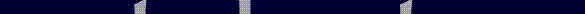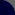Reviews in Undergraduate Research - Issue 1

 CP Violation and the Dominance of Matter } James Morris University of Arizona Communicated By: Dr. Elliott Cheu University of Arizona - Department of Physics

SUMMARY
The observable Universe reveals a clear dominance of matter over antimatter. If this asymmetry is consistent with the Universe as a whole, then a contradiction arises between observation and any naïve formulation of Big Bang theory. CP violation, violation of the combined C (charge conjugation) and P (parity) operators, is one of three necessary conditions of matter dominance theorized by Sakharov in 1967. Therefore, further study of CP violation is warranted to determine its role in the evolution of a matter-dominated Universe.

There are two ways by which violation might occur: direct and indirect. The first possibility is that physical eigenstates may be written as a linear combination of CP eigenstates - a small amount of the opposite CP eigenstate might cause CP violation. This violation from mixing of CP eigenstates is also called indirect CP violation. Independent of indirect violation, CP violation might also occur in the decay or direct CP violation. A famous experiment, which investigated the possibility of indirect CP violation, was conducted in 1964. Since then several experiments have been conducted to determine if CP violation also exists in the decay. This review summarizes the methodology of two such experiments.

Despite the progress made in understanding the nature of CP violation, the future of research of CP violation remains promising. Several proposed experiments involve determining the degree of CP violation in much more rare decays. I summarize one such future experiment of analyzing CP violation in these decays.

Finally, due to the rare occurrence of CP violation, future investigations will require high rate experiments with high efficiency detectors. To this end we test the efficiency of one such detector which may be used in such experiments. A high rate environment similar to that expected in proposed CP violating experiments was used to examine the detector's behavior. In particular, this detector was tested using electrons from KL decays (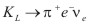). The high efficiency of this detector in this high rate environment is a testament to the future viability of this and similar devices in the investigation of CP violation.

MATTER DOMINANCE

The Naïve Big Bang
Big Bang Theory is widely accepted among the scientific community due to its empirical merits. For this reason, scientists have begun to establish particulars and study predictions of the theory. One prediction is that the early Universe was devoid of matter, containing only energy in the form of photons. As the Universe expanded, matter was able to form through processes such as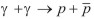(where g denotes a photon, p a particle, and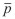an anti-particle). As can be seen, these processes produce equal numbers of particles and anti-particles. Naïve formulations of the Big Bang, which consider these processes alone, would result in a Universe that is matter/antimatter symmetric. However, we observe matter - not antimatter - out to 10Mpc (Dolgov, 1997), and strong arguments can be made in extending this observation to the Universe as a whole. Therefore, a contradiction arises between the small ratio of antimatter to matter, which current estimates place at 10-4, and a naïve formulation of the Big Bang.

Baryogenesis
The vast majority of matter is comprised of particles called baryons. Baryons, such as protons and neutrons, are particles with three quarks. There are two ways by which the baryon (or matter) asymmetry in our universe can be explained: either the abundance of baryons is the result of some set of initial conditions or the abundance came about by some physical mechanism. In 1967 Sakharov showed that at least three conditions are required for such a mechanism to exist (Sakharov, 1967). The conditions are

1. Baryon number violation
2. Thermodynamic Nonequilibrium
3. C and CP violation.

If some mechanism satisfies these three conditions, the baryon dominance we see today may be created from an early Universe which consisted mostly of energy. This is commonly referred to as baryogenesis. This review will concentrate on the CP violating condition of baryogenesis.

CP violation and Baryogenesis
Quantum mechanically, CP violation implies that the system is not symmetric under combined operations of charge conjugation (C) and parity inversion (P). The operation of charge conjugation exchanges particles with anti-particles, and the operation of parity inversion changes the signs of the coordinates. The combined operation of C and P relates the rates of particle transitions to anti-particle transitions. If CP were conserved, then the rate for a particular particle decay would be the same as that for the related anti-particle decay. If CP is violated, these transition rates would differ. For example, if the transition rate from anti-particle to particle is greater than the transition rate from particle to anti-particle, then matter creation would be preferred over antimatter creation.

NEUTRAL KAON AND CP CONSERVATION
CP violation was first discovered in decays of the K meson (a meson is a particle comprised of quark anti-quark pairs), also known as the kaon. Kaons have the unique propriety of being produced by the strong force and decaying via the weak force. This occurs because the kaon is the lightest particle with a strange quark. So, in order for the kaon to decay, its daughter particles cannot contain any strange quarks. However, the strong force preserves quark type. So, the kaon cannot decay via the strong force, but instead decays via the weak force, which allows quark flavor to change. Due to this phenomenon, one can write the weak eigenstates as superpositions of the strong eigenstates as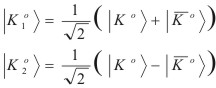where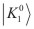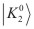are the weak eigenstates, and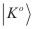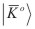the strong eigenstates. Furthermore, CP changesintoand vice versa. So, if we operate with CP onwe obtain an eigenvalue of +1, which is CP even. Similarly, if we operate with CP onwe obtain an eigenvalue of -1, which is CP odd. If CP is conserved, the weak eigenstates K1 and K2 above are identically the CP even and CP odd states, respectively

Because the K1 and the K2 are distinct under CP, their decay products must also be distinct. The K1 decays primarily into a state with two pi mesons (pions), which is even under CP, while the K2 decays primarily into a state with three pi mesons, which is odd under CP. The three pion state has a mass very close to the kaon. Because of this, there is very little extra energy, or phase space, available for the decay to occur. This causes the K2 to decay much less readily than the K1, which has significantly more phase space. In fact, the K2 has a lifetime approximately 600 times greater than the K1. For this reason the K1 is usually called KS and the K2 is called KL, where S and L designate the short and long lifetimes.

As mentioned, if CP is conserved, the KS should be equivalent to the K1 state and KL should be equivalent to the K2 state. Furthermore, if CP is conserved we expect that the K1 (or KS) should decay only into states such as KS ® 2p, and the K2 (or KL) should decay only into states such as KL ® 3p.

CRONIN-FITCH EXPERIMENT AND INDIRECT CP VIOLATION

An obvious method to determine if CP is conserved is to produce a well-defined CP state and observe its decays. If the initial state has different CP than the final state, then CP has been violated. In 1964 Cronin et al. performed this type of experiment (Christenson et al., 1964). They produced a beam of K mesons, which was allowed to travel a relatively long distance. The region near the end of the beam line contained mostly K2 mesons, K1 mesons having decayed away, so they expected to only see decays from K2 ® 3p. A signal for CP violation would be the appearance of K2 ® 2p decays in their detector.

The group used the Brookhaven AGS (Alternating Gradient Synchrotron) particle accelerator. A beam of protons bombarded a Be target producing an assortment of particles. Large magnets called sweeping magnets were used to remove charged particles so that a neutral kaon beam was produced. As the kaons proceeded down the beam line, they decayed, and the resulting daughter particles were detected by two detectors located at the end of the beam line. These detectors measured the momentum and positions of the daughter particles, which could be used to determine the momentum vector of the parent kaon particle. Because the detector only reconstructed two of the three daughter particles, the momentum vector of 3p decays reconstructs to a non-zero angle with respect to the original K2 beam. On the other hand, the momentum vector of 2p decays reconstructs coincident with the original K2 beam. A sophisticated computer program was used to determine the expected results of this angle if CP were conserved. This Monte Carlo simulation was compared to the results recorded from the experiment. Figure 1 shows the distribution of the angles between the reconstructed momentum vector and original K2 beam for all decays in a given mass region. Notice the significant peak above the Monte Carlo expectation, corresponding to zero angle decays. After subtracting backgrounds the group measured 45 ± 10 K2 ®p+ + p- decays of the 22,700 K2 decays - the first evidence of CP violation.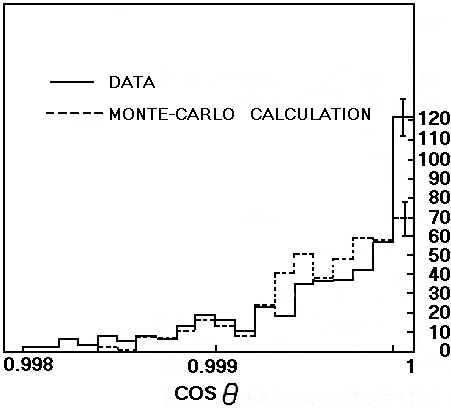Figure 1. Angular distribution of events with mass 490 < m* < 510 MeV

Indirect CP violation
The Cronin experiment demonstrated that CP is violated in weak decays. This discovery lead to the realization that the weak eigenstates are not the same as the CP eigenstates. In fact, one can write the weak eigenstates as admixtures of the CP eigenstates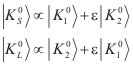In order for KL and Ks to be pure CP eigenstates the mixing parameter must go to zero, conserving CP. However, the Cronin experiment demonstrates that e must be nonzero, so the weak eigenstates are not pure eigenstates of CP but contain a small amount of the "wrong" CP value. For example, KS is not purely CP even but contains a small amount, e, of CP odd. Furthermore, we notice that e is independent of decay channel so we expect CP violating effects to have the same rate whether the decay mode is KL ®p° + p° or KL ®p+ + p-. Because this violation is due to mixed eigenstates, it is referred to as CP violations through mixing or indirect CP violation. The Cronin experiment obtained a parameter value of e » 2.3 X 10-3 which corresponds to 1 violation for every 500 decays.

DIRECT CP VIOLATION, NA48, AND KTeV

Direct CP Violation
The Cronin experiment showed that CP violation occurs when a state is composed of mixed CP eigenstates. The K®pp decay occurs through the K1 component. CP violation however, might occur in the direct decay of either K1 or K2, so that the K2 component might simply decay into two pions. This is called direct CP violation, the degree of which is measured by a parameter e'. Note that this violation does not appeal to an admixture of CP states but occurs directly in the decay. This is why direct CP violations are sometimes referred to as violations in the decay. So there are two means by which CP may be violated. Either the K1 component of the KL decays into two pions (indirect CP violation). Or, the KL decays into two pions via the K2 state (direct CP violation). These possibilities are shown below: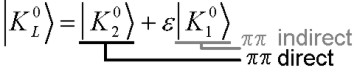e'/e and the Double Ratio
Although indirect CP violation manifests itself in the KL ®2p° decay, CP violation also occurs in other decay modes such as KL ®p+ + p-. If all CP violating effects were due to indirect CP violation, which is decay channel independent, then the rates for different decay modes would be the same. So if one were to compare the indirect violation in two different modes, and find different rates, this must be due to a direct CP violation.

One method to search for direct CP violation is to compare K ®p°p° decays to K®p+ + p- decays. The ratio e'/e can be determined from the following ratio of CP violating rates: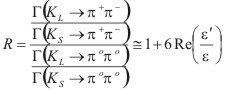where G designates the rate of occurrence of the decay (Alavi-Harati, 1999). Notice that the numerator and denominator of this ratio are both measures of indirect CP violation, where we expect the KL violations to occur about once in every 500 decays. If direct CP violation does not exist, we would expect the double ratio to equal unity, forcing the e'/e term to vanish as expected. However, if CP violations are decay channel dependent, the ratio R will deviate from unity resulting in a nonzero e'/e , evidence of direct CP violation.

NA48 and KTeV
Searches for direct CP violation have been performed since the Cronin experiment, but it was not until the last decade that experiments have obtained statistically significant results of direct violation. The difficulty in determining e'/e is due to the fact that direct CP violation is expected to be much smaller than indirect CP violation. About five years ago two promising experiments set out with a goal of obtaining a definitive value of e'/e.

The determination of the double ratio amounts to essentially a counting experiment. The two CP violating rates are just proportional to the number of particles from each decay mode within a certain decay volume. The difficulty in this measurement lies in efficiently determining the decay rates while subtracting errors from backgrounds and accidentals. One advantage of this measurement is that a number of systematic effects will cancel in the double ratio. So, it is important to collect all four decay modes simultaneously. As mentioned, the Cronin experiment recorded about 22,000 events of which 45 ± 9 were indirect CP violating. However, to measure e'/e to the desired precision nearly 20 million indirect CP violating decays have to be collected (Alavi-Harati, 1999).

In 1997, a group in experiment NA48 at CERN took data used to analyze e'/e. In three separate runs that lasted months at a time, NA48 was able to record over ten million events per run. This large number of events is possible due to the high rates produced by today's particle accelerators and the high efficiency of modern particle detectors. NA48 utilized a liquid krypton calorimeter to measure energy deposition and a bent crystal to extract protons onto a KS target. Such a setup is necessary to produce a simultaneous collinear KS beam, which is required to eliminate errors in the double ratio. After three years of taking data, NA48 reported a value of (15.3 ± 2.6) X10-4 for e'/e (Lai, 2001).

A second group under the KTeV collaboration used the Tevatron at Fermilab in investigation of e'/e. The setup and general methods are similar with NA48, but various components are constructed differently. For example, KTeV uses a pure CsI crystal calorimeter to measure energy deposition. To create the collinear KS beam, KTeV uses a unique property of kaons whereby a KL turns into a KS when passed through a thin slab of matter. This is due to the strong interactions of kaons with protons and neutrons when passing through matter. (This regeneration process was also studied in the Cronin experiment.) Every second, 200 billion protons hit a beryllium target similar to the one used in the Cronin experiment (Cheu, 1997). An extremely high rate trigger determines 53 million times a second which events to use in data analysis. This corresponds to 10 MB of data every second, which over the KTeV running period, results on the order of 100 TB of data collected. After analyzing only one eighth of their data, the KTeV group was able to attain a statistically significant result much larger than the theoretical expectations of (4-10) X 10-4 (Ellis, 1976 and Ciuchini,1997). Combined with later data, which amounts to about one-half of the total KTeV data, resulted in a value for Re (e'/e) of (20.7 ± 2.8) X 10-4.

Overall Average
After averaging all recent direct CP violation experiments, one obtains a world average of (17.2 ± 1.8) X 10-4 as shown in Figure 2. This result conclusively shows that direct CP violation exists, a new form of matter/antimatter asymmetry. Note that the small value of this result signifies that direct CP violation is much smaller than indirect violation.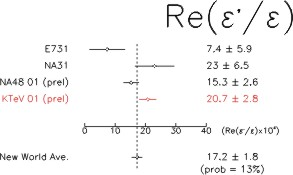Figure 2. Values reported by various experiments for e'/e and the world average.

THE FUTURE OF CP VIOLATION

KOPIO
The KTeV and NA48 experiments combined with the Cronin experiment definitively show that there are two types of CP violation: direct and indirect. However, theoretical calculations of e'/e are very challenging because of our lack of understanding of the strong force. To achieve a better understanding of CP violation, an investigation of other decay channels is necessary. The KOPIO experiment using the Brookhaven AGS - the same used by Cronin et al. - will attempt to study one of the more rare decay channels. In particular, the decay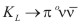has some interesting features which are worthy of investigation. In this decay the amplitude for the indirect CP violating component is actually expected to be much less than the amplitude for the direct component. In addition, the theoretical uncertainties for this decay mode are very small because the final state does not include any hadronic particles such as pions, which are theoretically difficult to handle because they involve the strong interaction. So, measurement of this decay mode would allow a much better understanding of direct CP violation (Littenberg, 1989). This decay occurs about once in every 100 billion decays, making it particularly difficult to study. Experimentally, the final state consisting of two photons (from the p°) and two neutrinos is difficult to reconstruct. Because the neutrinos only interact via the weak interaction, they will remain undetected in the experimental apparatus, so the challenge is locating two isolated photons and nothing else in the detector. Given the very high rate of backgrounds expected in this experiment, the KOPIO group faces a number of challenging tests in searching for CP violation in rare decays. However, a positive result from this group will help to further our understanding of CP violating effects.

New Detectors
The next generation of particle detectors and the next batch of CP violation experiments will continue to push the limits of our current technology. More than likely, the next generation of experiments will require much more precise and accurate detectors with the ability to obtain high precision under extreme conditions. One such detector has been examined under the KTeV project at the University of Arizona.

This detector is composed of alternating bars of quartz and uranium, and is situated directly in the beam line. This is a unique region for detectors, which are usually adjacent to the beam line where the interaction rate is much lower. A detector residing in the beam line is well-suited for experiments like KOPIO, where there are many background processes to a decay such as. Such backgrounds can fake a signal if some of the daughter particles continue down the beam line and remain undetected. Placing such a detector in the beam line will reduce the probability for such backgrounds. The quartz-uranium detector operates by measuring the scintillation light resulting from interactions of charged particles with the quartz bars. Photons also can be detected as they shower in the heavier uranium, producing a multitude of charged and neutral particles. The measure of the resulting scintillation light is proportional to the amount of energy deposited.

In analyzing the detector's efficiency, KL decays were reconstructed in the KTeV detector. Since the environment in the KTeV detector is similar to that expected in future experiments, this analysis should indicate how our quartz-uranium detector will perform in an experiment such as KOPIO. The electrons from KL decays are identified using the KTeV detector, which is upstream of our quartz-uranium detector. Then, with the particles' identified, we test the ability of the quartz-uranium detector to correctly identify them. Despite the high rate environment of over 1 MHz, the detector is still able to properly identify particles correctly with very high efficiency (in this case electrons). In the KOPIO experiment, one is more interested in photons, but since electron and photon interactions are expected to be similar in our detector, these results indicate how well our quartz-uranium detector should perform for photons. Our result is no misidentified electrons from more than 40,000 events used in analysis. This places an upper limit of the detector's inefficiency at 5.0 X 10-5 at the 90% confidence level. This high efficiency signifies a promising future for tomorrow's detectors in future experiments such as KOPIO.

CONCLUSIONS

CP Violation Today
Although indirect CP violation was first discovered by Cronin et al. in 1964, the search for direct CP violation is a fairly recent endeavor. It was only in the last decade that a high enough precision has been reached to verify direct CP violation. Direct CP violation has been discovered and, as expected, it has a much smaller effect than indirect violation. Furthermore, CP violation is still in the early stages of understanding. We await experiments such as KOPIO to obtain a new outlook on direct CP violation. An interesting possibility in these experiments is the discovery of physics beyond the standard model. The standard model is the set of current theoretical facts, confirmed from experiment, which govern particle physics. The standard model is able to make certain theoretical predictions which experiment will confirm or deny. Thus far the standard model is consistent with experiment; however, if an inconsistency is discovered this will give rise to go beyond current physics and establish a new theory. CP violation is a phenomenon which may provide this avenue away from the standard model.

Baryogenesis Revisited
Over the past three decades a preference of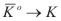over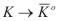has been established through CP violation. Thus one of the Sakarov conditions is now known to exist. Note however, that although CP violation exists, CP violation in weak decays cannot account for the degree of matter/antimatter asymmetry in the Universe. In fact CP violation in weak decays is too small by a large factor to account for baryogenesis. Future experiments will search for CP violation elsewhere, which is believed to manifest itself B meson system also; however current theory points to no resolution even under this consideration. To resolve this issue we might need to appeal to CP violation in models currently beyond the standard model and outside of the realm of current experimental confirmation. It may be that only under considerations of Supersymmetric models (SUSY) or models at the level of Grand Unified Theories (GUT) will the riddle of matter dominance finally be solved.

ABOUT THE AUTHOR
James Morris is an undergraduate senior attending the University of Arizona. He plans on attending a doctorate program in physics. He aspires to become a professor of physics at the university level and to contribute to current research in the field. James assists the high energy department at the University of Arizona. In particular he is testing the efficiency of next-generation particle detectors for KTeV which may be used in future experiments involving CP violation. The detector under analysis is a quartz-uranium detector which has the uncommon feature of residing directly in the beam line. Such a region is rare for particle detectors, which usually reside adjacent to the beam line in detecting electromagnetic showers. This region exposes the detector to a high rate environment on the order of 1 MHz. And this fact combined with the higher precision required by future experiments creates the need for a high efficiency. Despite these circumstances the detector is still able to attain high efficiency.

ACKNOWLEDGEMENTS
I would like to thank the High Energy group at the University of Arizona for their assistance and use of their resources. I am particularly grateful to Dr. Elliott Cheu for his assistance and guidance on this project.

FURTHER READING

Baym, G. (1973). Lectures on Quantum Mechanics. (Menlo Park, CA: Benjamin/Cummings
Publishing).

Bigi, I.I., and Sanda, A. I. (2000). CP Violation. (New York, NY: Cambridge University Press).

Branco, G. C., Lavoura, L., and Silva, P.J. (1999). CP Violation. (New York, NY: Oxford University Press).

Cahn, R.N., and Goldhaber, G. (1995). The Experimental Foundations of Particle Physics. (New York, NY: Cambridge University Press).

REFERENCES

Alavi-Harati, A., et al. (1999). Observation of direct CP Violation In K(S,L) --> PI PI Decays. Phys. Rev. Lett. 83, 22.

Cheu, E., et al. (1997). Proposal to continue the study of direct CP violation and rare decay processes in KTeV in 1999. FERMILAB-P-0799

Christenson, J.H., et al. (1964). Evidence for the 2p decay of the K2o meson. Physical Review Letters. 13, 138-140.

Cicuchini, M. (1997). Epsilon-Prime / Epsilon: Three Years Later. Nucl.Phys.Proc.Suppl. 59. 149-156.

Dolgov, A.D. (1997). Baryogenesis, 30 Years After. Presented at 25th ITEP Winter School of Physics TAC-1997-024. [HEP-PH 9707419]

Ellis, J., Gaillard, M. K., and Nanopoulos, D. V. (1976). Lefthanded Currents And CP Violation. Nucl.Phys. B109; Gilman, F. J. and Wise M. B. The Delta I = 1/2 Rule and Violation of CP in the Six Quark Model.Phys. Rev. Lett. B83. 83.

Lai, A. (2001). A Precise Measurement of the Direct CP Violation Parameter RE (Epsilon-Prime / Epsilon). Eur. Phys. J. C22. 231-254.

Littenberg L. (1989) The CP Violating Decay K0(L) ---> PI0 Neutrino Anti-Neutrino. Phys. Rev. D39. 3322-3324

Longair, M. S. (1998). Galaxy Formation. (Heidelberg, Berlin: Springer) pp. 231

Sakharov, A.D. (1967). Violation of CP Invariance, C Asymmetry, and Baryon Asymmetry of the Universe. Pisma Zh.Eksp.Teor.Fiz. 5, 32-35.

Steigman, G. (1976). Observational tests of antimatter cosmologies. Annual review of astronomy and astrophysics. 14, 339-372.

 RUR Issue - 1